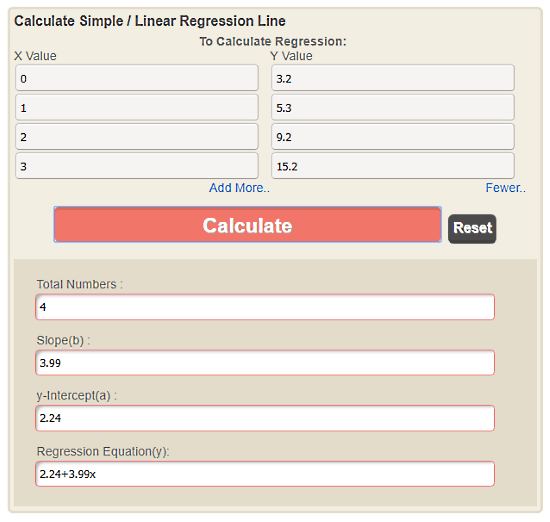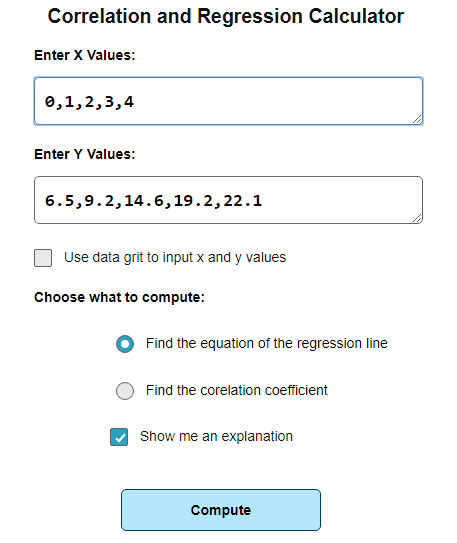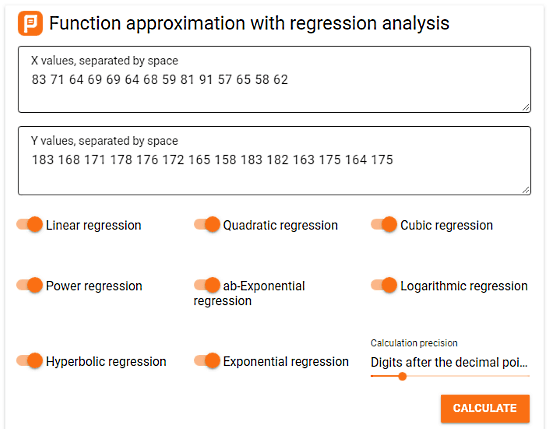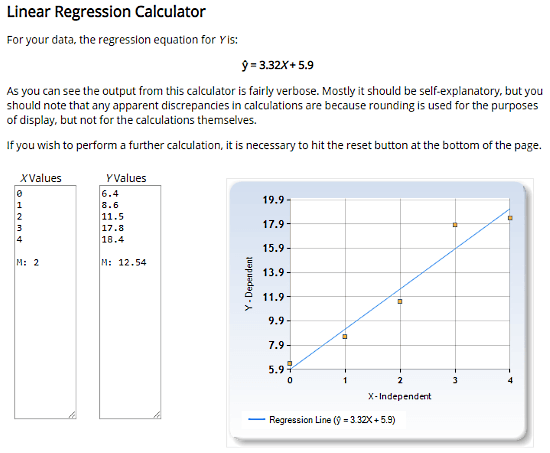Editor Ratings:
User Ratings:
[Total: 0 Average: 0]

Linear regression is an approach to model the relationship between one scalar(dependent) variable/response and one explanatory(independent) variable. It is defined by the following formula:

y = c + b * x

where,

• y = estimated dependent variable
• c = constant
• b = regression coefficient
• x = score on independent variable

Linear regression has useful application in many sectors such as engineering research, marketing, weather data analysis to many more.

This article covers 8 free websites where you can calculate linear regression online. These websites are arranged in increasing order of functionalities. Most of these websites also plot a graph of the regression line. Some of those graphs are interactive; you can trace the values on the graph. Moreover, some websites also offer a step-by-step explanation of the solution. So, let’s check out these websites one by one.

Also read: Top Free Exponential Equation Calculator Websites

## EasyCalculation.comEasyCalculation offers a very simple and straightforward tool to calculate linear regression. Here, you get two columns for the input; one for the values of x values and one for the values of y. By default, you get two rows in each column although you can add as many as you need. When you calculate the linear regression, this calculator gives you Total Numbers, Slope, and y-Intercept along with Regression Equation in the output. You can also add this calculator to a website/webpage. It gives you an option to enter dimensions and generate the embed code to add this calculator to your website.

You can try this simple linear regression calculator here.

## Acula.comAcula is a collection of online calculators and conversion tools. It has a simple calculator to calculate linear regression online. This calculator has a single text box for input data. Here, you have to enter a single value of x and y in each line separated by a comma. You can as many values as you want and submit that data to calculate the linear regression. In the output, it gives you Sample size, Mean x, Mean y, Intercept, Slope, and regression line equation. It also plots the regression equation line on a graph which you can download as a PNG image from the right-click context menu. Moreover, this calculator also has an option to estimate the value of y for any given value of x using the resulted regression equation.

Give this online linear regression calculator a try here.GraphPad is a software company that creates software for the scientific community. Although many of their products are not free, this website does offers some handy tools for free. One of these tools is QuickCalcs. This tool has a collection of calculators including a linear regression calculator under Statistical Distributions. In this calculator, you get 100 rows for the values of x and y, and you can label them accordingly. You can enter your values to this tool and calculate the linear regression. In the output, it gives you Best-fit values95% Confidence IntervalsGoodness of FitIs slope significantly non-zero?, and Data. These fields contain slope, y-intercept, x-intercept, R square, Sy.x, F, P valuedeviation from horizontal, number of XY pairs along with the regression equation. Moreover, it also plots the regression equation on a graph and you can download that graph as an image file.

Calculate linear regression online with GraphPad here.

## MathPortal.orgMathPortal is a free website filled with math formulas, math lessons, math tests, and calculators. It has a correlation and regression calculator which you can use to calculate linear regression online. This calculator has two text boxes for the values of x and y. You can type the values of x and y in these text box or switch to a data grid and enter your input there. Then, you can find the equation of the regression line or find the correlation coefficient for the input data. Below these options, there is a checkbox to include the explanation in the output. Along with the selected computer results, this calculator gives you the regression line equation along a regression line graph.

You can try this online linear regression calculator here.

## PlanatCalc.comThe next website to calculate linear regression online on this list is PlanetCalc. This website has a multipurpose calculator where you can easily calculate the linear regression. Apart from the Linear Regression, here you can also calculate Quadratic Regression, Cubic Regression, Power Regression, ab-Exponential Regression, Logarithmic Regression, Hyperbolic Regression, and Exponential Regression. You can also set the decimal digit precision value anywhere from 0 to 20. In the results, this calculator gives detailed results with output for each pair of x and y values. It also plots an interactive graph where you can see the values of x, y, and regression. You can save the output results to your PlanetCalc account, share them online on social media or via mail. You can generate code for HTML or WordPress to embed the results on a web page.

Calculate regression online with PlanetCalc here.

## GoodCalculators.comGoodCalculators is a collection of free online calculators covering a variety of fields from mathematics, engineering, logistics, sale, finance, HRM to health. It has a powerful linear regression calculator where you can enter the values of x and y, and calculate the regression equation. In this calculator, you can one by one input as many pairs of x and y values as you want. When you calculate the results for the input values, this calculator gives you the Sample size, Mean x, Mean y, Slope, Intercept, Regression equation, and correlation coefficient in the output. It also shows you all the regression data (x*y, x2, y2, etc.) and calculates their mean as well along with an interactive graph. On top of that, you can also see a step-by-step explanation of the results here.

You can try this online linear regression calculator here.

## SocSciStatistics.comSocSciStatistics offers free resources for statistics in social science. It has a quick linear regression calculator where you can calculate linear regression online. In this calculator, you get two separate text boxes to enter the values of x and y. It also has another textbox for Estimate but that is not for linear regression. So, you can simply enter the values here and calculate linear regression. In the results, it gives you the regression equation at the top accompanied by the regression line graph. Below that, it has calculation summary that contains sum of x, sum of y, mean x, mean y, sum of squares, sum of products, regression equation, etc. for your data. You can copy-paste the results to a text editor and save them for later use.

Calculate linear regression with SocSciStatistics here.

## Desmos.comDesmos is an online multiple purpose tool where you can graph functions, plot data, evaluate equations, explore transformations, and much more. Here, you can create an expression using an equation and use that expression to calculate the correspondings. This tool already has such an expression for linear regression. You can simply type your data here and calculate linear regression. As you calculate the linear regression, it marks all the values on the graph and plots the regression line. The graph here is fully interactive; you can trace the regression the line with the mouse cursor to see value at any point. You can displace points on the graph as well. Apart from the graph, this tool gives you the values of various regression terms. You can get the regression equation by arranges these terms by expression.

Calculate linear regression and plot graph with Desmos here.

### Closing Words:

These are the 8 free websites to calculate linear regression online. Using any of these websites, you can quickly calculate the linear regression equation for your data. Give them a try and share your opinion with us in the comments.

 Editor Ratings: User Ratings:[Total: 0 Average: 0] Free/Paid: Free Tags: equation